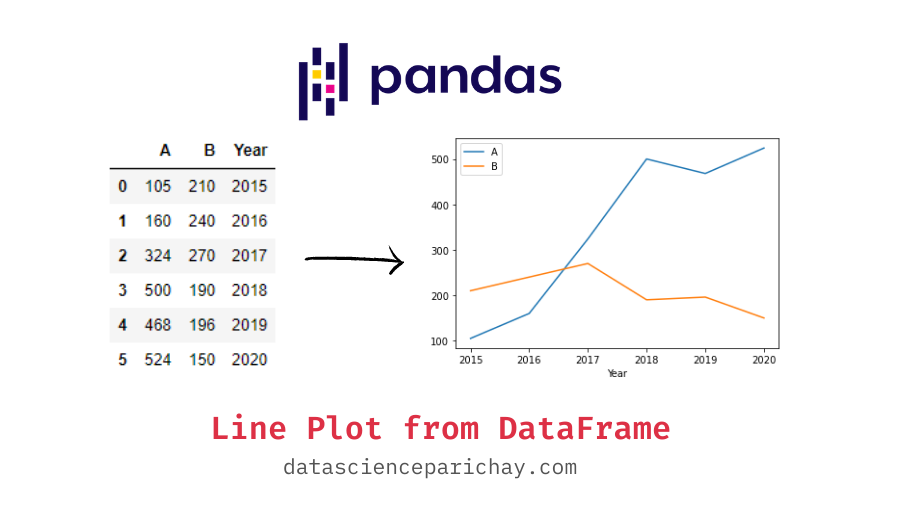# Create a Line Plot from Pandas DataFrame

In this tutorial, we’ll look at how to create a line plot from a pandas dataframe.

To create a line plot from dataframe columns in use the pandas `plot.line()` function or the pandas `plot()` function with `kind='line'`. The following is the syntax:

```ax = df.plot.line(x, y)
# or you can use
ax = df.plot(kind='line')```

Here, x is the column name or column number of the values on the x coordinate, and y is the column name or column number of the values on the y coordinate.

Under the hood, the `df.plot.line()` function creates a matplotlib line plot and returns it. You can also use the matplotlib library to create line plots by passing the dataframe column values as input.

Let’s look at some examples creating a line plot directly from pandas dataframe. First, we’ll create a dataframe that we’ll be using throughout this tutorial.

```import pandas as pd

# dataframe of sales of product A and B from 2015 to 2020
df = pd.DataFrame({
"A": [105, 160, 324, 500, 468, 524],
"B": [210, 240, 270, 190, 196, 150],
"Year": [2015, 2016, 2017, 2018, 2019, 2020]
})

print(df)```

Output:

```     A    B  Year
0  105  210  2015
1  160  240  2016
2  324  270  2017
3  500  190  2018
4  468  196  2019
5  524  150  2020```

The dataframe contains the information on units sold of products A and B by a retailer from 2015 to 2020.

If you use `df.plot.line()` without any arguments, it plots all the numerical columns as separate lines.

📚 Data Science Programs By Skill Level

Introductory

Intermediate ⭐⭐⭐

🔎 Find Data Science Programs 👨‍💻 111,889 already enrolled

Disclaimer: Data Science Parichay is reader supported. When you purchase a course through a link on this site, we may earn a small commission at no additional cost to you. Earned commissions help support this website and its team of writers.

`ax = df.plot.line()`

Output:

You can see that all the numerical columns in the dataframe are plotted as a line each in the resulting plot. You can specify the column to be used as the x-axis values by passing its name to the `x` parameter or setting that column as the index of the dataframe.

`ax = df.plot.line(x='Year')`

Output:

Here, we passed the “Year” to the `x` parameter to use it as the x-axis values. The other numerical columns, “A” and “B” were plotted as a line each.

If you only want to plot just one column as a line on the y-axis, pass that column’s name to the `y` parameter.

`ax = df.plot.line(x='Year', y='A')`

Output:

Here, we passed “A” to the `y` parameter and “Year” to the `x` parameter resulting in a line plot with only the sales of product A against the year.

You can also plot each numerical column in the dataframe on separate subplots. For this, pass `subplots=True` to the plot function.

`ax = df.plot.line(x='Year', subplots=True)`

Output:

You can see that have separate subplots for sales of A and B.

For more on the line plot function in pandas, refer to its documentation.

With this, we come to the end of this tutorial. The code examples and results presented in this tutorial have been implemented in a Jupyter Notebook with a python (version 3.8.3) kernel having pandas version 1.0.5

•